Home    Flowchart construction    Documentation    Examples    Links    F.A.Q.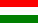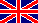# Tutorial on using the distillation and loss operations

## Example - model of ethanol production by fermentation using this flowchart editor / process simulator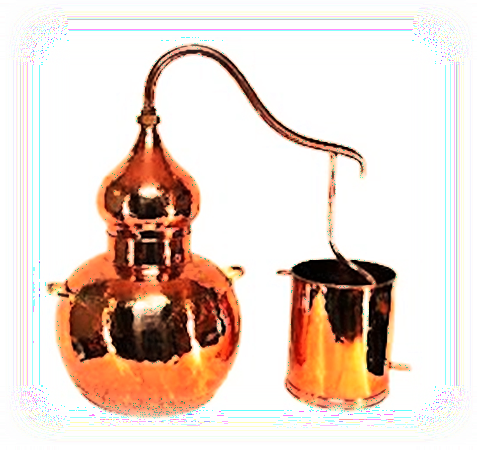## Before you continue...

...you should have a basic understanding of the following:

## Topic and recipe of example project

Produce ethanol from sugar by fermentation

Dissolve 30 kg of sugar in 100 litres of aqueous 2.9 g/l sodium citrate buffer solution. Add some fermentation culture to it and let the formed CO2 leave into the air.

Then distill off the formed ethanol from the mixture.

Tip: try to follow this tutorial with the flowchart editor using example project: Ethanol production by fermentation!

## Chemical reactions: transformation of compounds

The main point of the operation "reaction" is to define transformation of compounds. Chemical reactions, microbiological transformations - these are the same. One point is common: the principle of mass conservation must be satisfied, i.e. as many mass reacts ("disappears" from the system) so many mass must form ("appear" in the system). The program forces it consequently.

Note: this program version does not requires total knowledge about reaction mechanisms as well as it does not requires the chemical equations coefficients. Even complex reaction systems can be handled either "careless" or precise. The main point is the mass balance.

Reactants and products should be defined this way:
• You can use the %C unit here, too, it makes projects more robust to scale changes. But in case of more complex systems the use of mass units could be a better choice because keeping the mass balance is easier with it.
• the role of the component must be selected in the type column (i.e. it reacts or it forms, and that the component should be considered as key compound or not).

Let's see an example (as in the title): Produce ethanol from sugar by fermentation.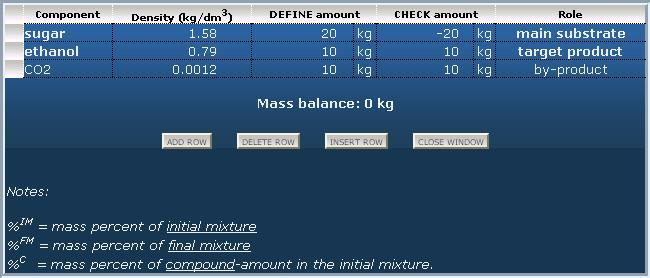Another example: Produce the sodium citrate mentioned in the recipe by reaction of sodium hydrogen carbonate and citric acid.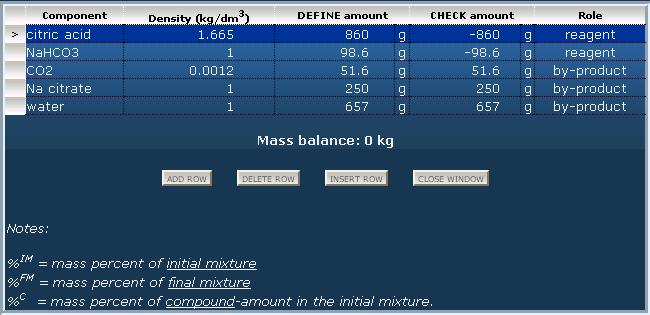## Loss by reactions: handling of gaseous compounds

There are reactions where gaseous compounds form (in our example carbon dioxid), and there are reactions where gaseous reagents are used (e.g. hydrogenation). Excess gaseous reagents and useless gaseous by-products may be released out into the air, and for this kind of loss can be used the "loss" operation. Before this an output material must be definied for summarizing losses (e.g. "lost compounds into the air" or "exhaust gases into the air").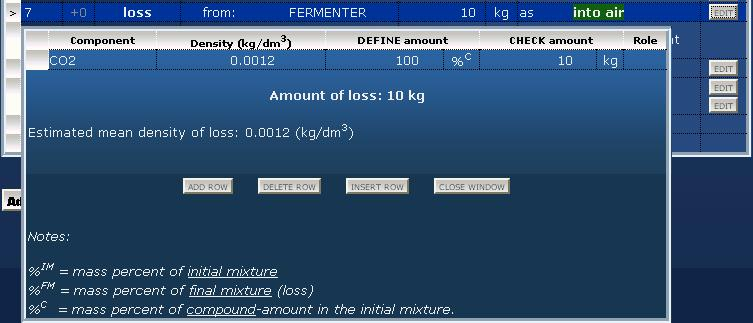Another solution is the absorption of the formed gases in an absorber equipment. (e.g. the formed hidrochloric acid gas is conducted to dilute sodium hydroxide solution.)

Page top        Home

The Web

Useful tools

Chemical Equations Balancer
Solving of chemical equations, automatic determination of stochiometric coefficients.

Chemical Reaction Calculator
Calculate the balanced equation and equilibrium constant.

Reaction Balancing & mol.weight tool
Reaction Balancing and Molecular Weight Calculation Tool

Yield (%) calculator
Reaction yield (%) calculation from masses and molecular weights

Chemical Equations Balancer
Solving of chemical equations, automatic determination of stochiometric coefficients.

Chemical Reaction Calculator
Calculate the balanced equation and equilibrium constant.

Reaction Balancing & mol.weight tool
Reaction Balancing and Molecular Weight Calculation Tool

Yield (%) calculator
Reaction yield (%) calculation from masses and molecular weights

process-flow-diagram.com    --    generate chemical technological flowsheets based on material flow calculations